# How to change or convert number to text in Excel?

If you keep long and short numbers in Excel spreadsheets, at some point you will likely need to convert them to text for usage in other applications. Changing digits that have been saved as numbers to text could be done for a variety of reasons.

Excel is a powerful tool that can be used for a variety of purposes, including the entry and analysis of data, the creation of dashboards and reports, and even database management.

Although the majority of the pre-installed features and default settings are designed to be helpful to users and to save them time, there are instances when you may find that you wish to make some adjustments to how things work.

In this tutorial, we will show you to convert numbers to text using some method.

## Using the Text Function

Keeping track of numeric values in Excel is typically a good idea because those values can be plugged into formulas that carry out computations based on numbers. Having said that, there are several instances in which translating numbers to text makes perfect sense.

Converting numbers to text using a specific number format can be accomplished with the help of the TEXT function. Absence of the TEXT function will result in the loss of the number formatting.

### Step 1

In our example, we have Numbers in our Excel sheet as shown in below given image. We will change the numbers to text.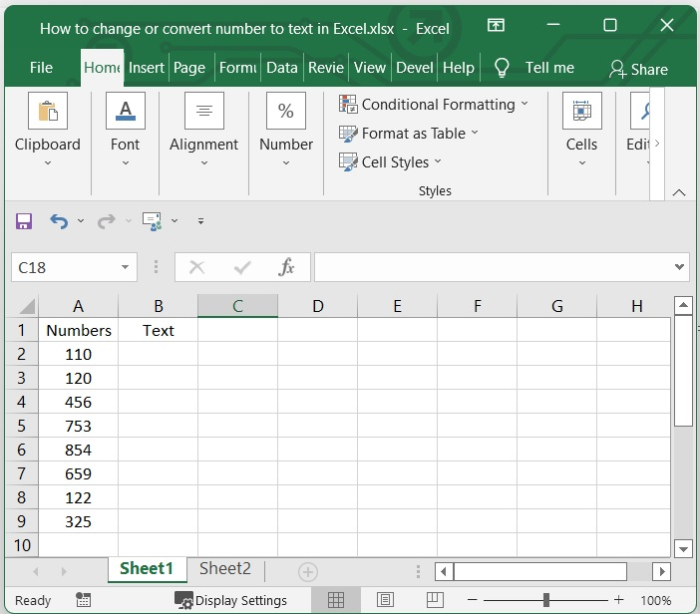### Step 2

Then select one cell and add the below given formula in formula bar. In our example, we have selected B2 cell and added the below given formula.

=TEXT(A2,"0")


After adding the formula, press enter. See the below given image.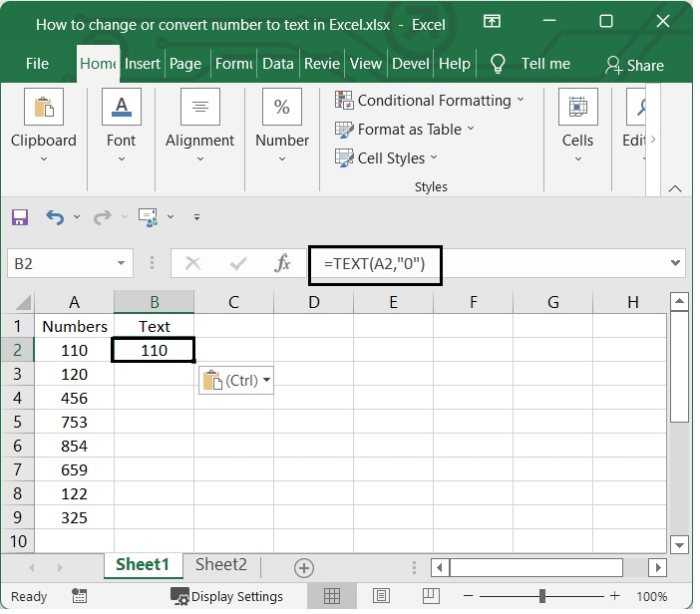### Step 3

After that, choose B2, and drag the fill handle over the cells that you want to apply this formula to. Take a look at the following screenshot.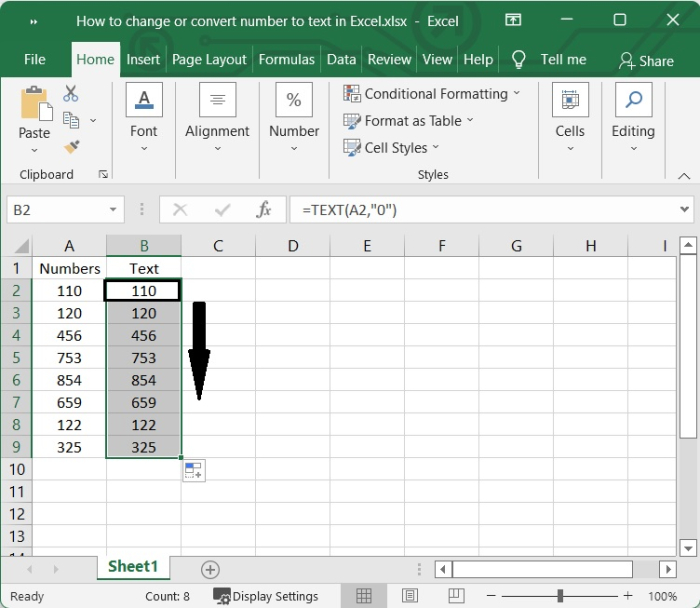## Using the Format Cell Option

The Format Cells command in Microsoft Excel has the additional capability of converting integers to text.

### Step 1

Let’s consider the above given example. To convert the numbers in the cell to text, select the numbers. See the image given below.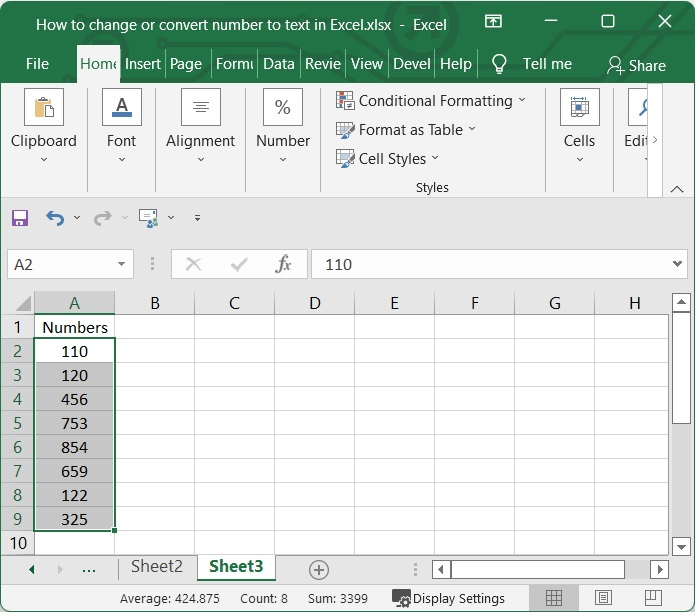### Step 2

Then right-click the range you want to change, and then choose Format Cells from the menu that comes up. See the image.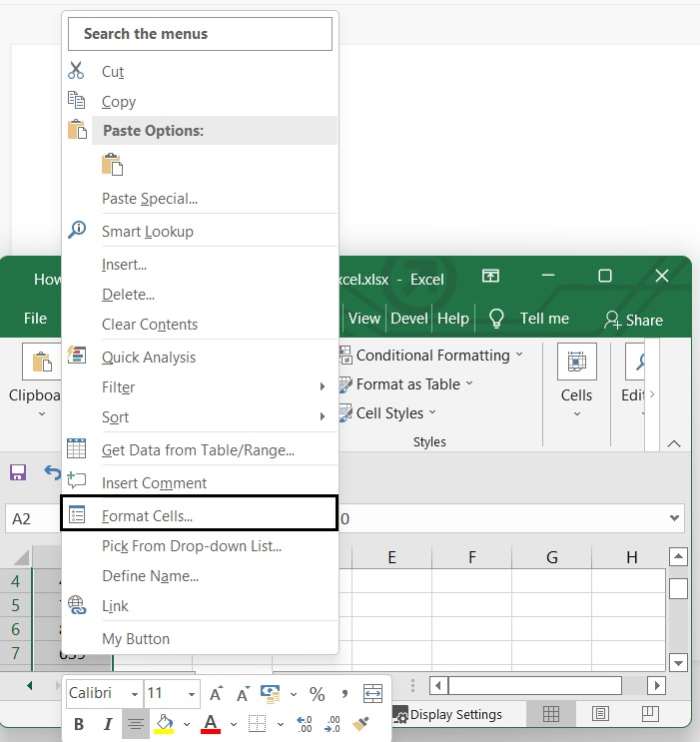### Step 3

In the Format Cells dialogue box, choose Text in the Category box under the Number tab. Then click the OK button. See the picture.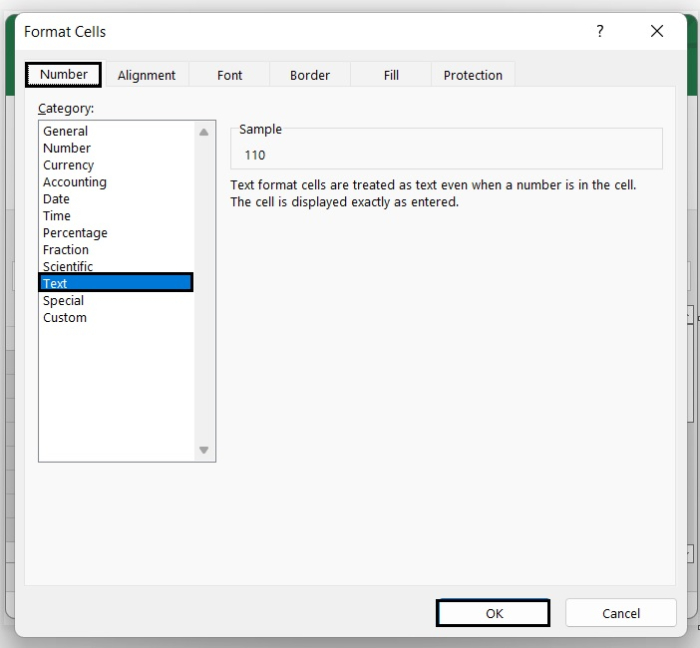Here's a quick tip for when you are manually inputting data into Excel and don't want the numbers to convert to text format.

To convert the numbers to text, put an apostrophe in front of the number. See the below given image.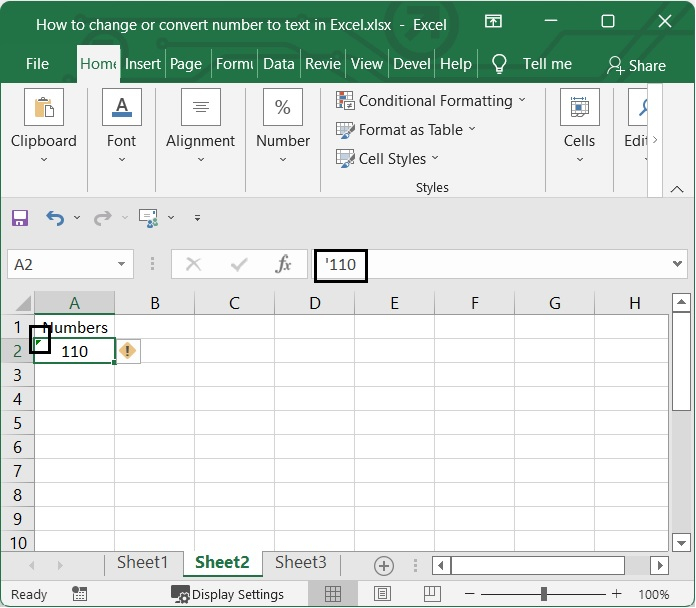## Conclusion

In this tutorial, we explained two methods that you can use to convert numbers to text in Excel.

Updated on: 06-Feb-2023

1K+ Views